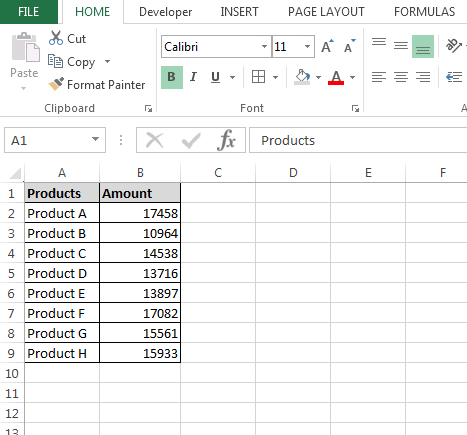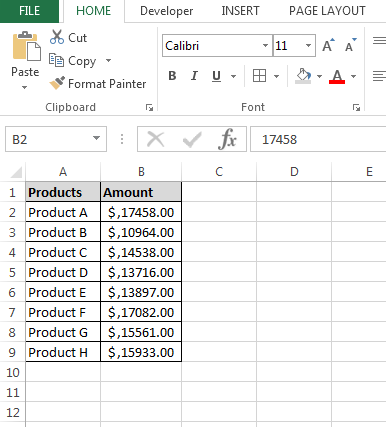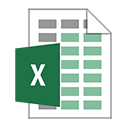# Accounting Number Format through VBA Formatting

Let’s take an example to understand how and where we can format the cell for accounting.

We have product’s amount data in range A1:B9 in which column A contains Product name and column B contains amount of it. We want to format the amount range as accounting. The format puts dollar sign in the left and add two decimals to the number. This format is meant to display money.To write the macro, follow below given steps:-

• Open VBA Page press the key Alt+F11.
• Insert a module.
• Write the below mentioned code:

Sub FormatAccounting()

Dim Sh As Worksheet

Set Sh = ThisWorkbook.Sheets(1)

Sh.Range("B2:B9").NumberFormat = "_(\$*#,##0.00_);_(\$*(#,##0.00);_(\$*""_""??_);_(@_)"

End Sub

Code Explanation:-

First we have given the subject for the code, then we had defined all the variables after that we had defined the range where we want to put the accounting format.

When you will run the Macro by pressing the key F5, you will get the desired result in Microsoft Excel.@ www.excelforum one of the user has asked about the similar question:-

I have this code but can’t seem to figure out how I can put a dollar sign in front. Code below does not put a \$ sign in front of this value 2220.45-. Would like it to display as \$2220.45-